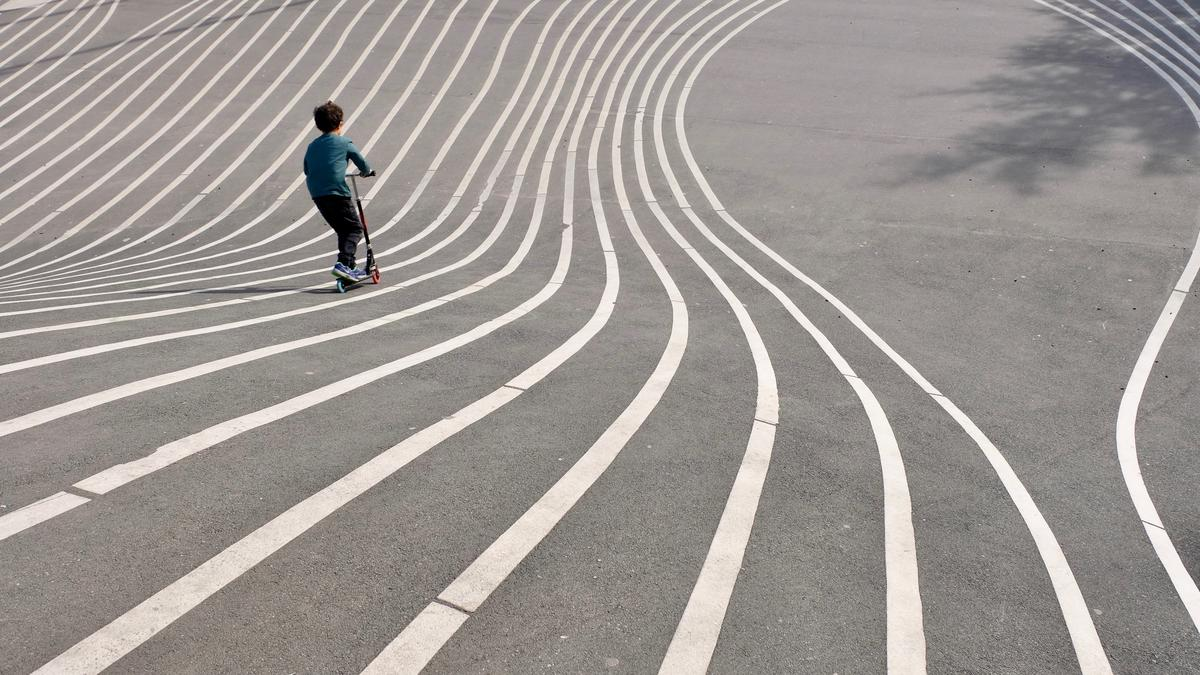# Why ggplot2 geom_line() does not draw lines?Image credit: Sam Poullain on Unsplash

# TL;DR

You want to link all data points and draw a line using the `geom_line()` from the `package:ggplot2` but it does not show the lines. The answer is that the X variable is a factor type.

# Welcome

This is a question from my student at the Singapore Management University who is studying data analytics under me. So this post is a record for future reference. I hope you will find it useful.

# geom_line() does not generate lines

Let’s first load the data we will use to draw the lines. This is a subset of housing price data in the US.

``````df <- read.csv("house.csv")
str(df)
``````
``````## 'data.frame':    1000 obs. of  9 variables:
##  \$ zip           : int  501 501 501 501 501 501 501 501 501 501 ...
##  \$ StateName     : chr  "NY" "NY" "NY" "NY" ...
##  \$ State         : chr  "NY" "NY" "NY" "NY" ...
##  \$ City          : chr  "Holtsville" "Holtsville" "Holtsville" "Holtsville" ...
##  \$ Metro         : chr  "New York-Newark-Jersey City" "New York-Newark-Jersey City" "New York-Newark-Jersey City" "New York-Newark-Jersey City" ...
##  \$ CountyName    : chr  "Suffolk County" "Suffolk County" "Suffolk County" "Suffolk County" ...
##  \$ date          : chr  "X2012.02.29" "X2012.03.31" "X2012.04.30" "X2012.05.31" ...
##  \$ housing_index : int  79658 79797 79934 79978 80197 80390 81135 81417 81815 81788 ...
##  \$ housing_growth: num  -0.000778 0.001745 0.001717 0.00055 0.002738 ...
``````

Now we try to plot the average housing growth rate for all years. The year information is contained in the variable `date`. So we first extract the year which is from the 2nd to the 5th characters.

``````df\$year <- substr(df\$date, 2, 5)  # extract year
``````

Then we draw the plot using ggplot2.

``````library(tidyverse)
library(plotly)
p <-df %>%
group_by(year) %>%
mutate(mean_growth = mean(housing_growth, na.rm = T)) %>%
slice(1) %>%
ungroup() %>%
ggplot(aes(x = year)) +
geom_point(aes(y = mean_growth, color = "average growth")) +
geom_line(aes(y = mean_growth, color = "average growth")) +
theme(axis.text.x = element_text(angle = 90, vjust = 0.5, hjust=1)) +
scale_y_continuous(labels = scales::percent) +
ggtitle("Average growth by a U.S. State, 2000-2021") +
labs(x="Year", y="Growth")
ggplotly(p)
``````

As you can see in the above plot, only the data points are shown but there is no line. Let’s check the data type for `year`.

``````str(df\$year)
``````
``````##  chr [1:1000] "2012" "2012" "2012" "2012" "2012" "2012" "2012" "2012" ...
``````

It is a character data type. ggplot2 will automatically recognize the character data type as a factor data type and each level of the factor (ie, each year) will be regarded as a group. geom_line() will only link all points which belong to the same group. So it will only link points which belong to the group of 2000 or the group of 2021. However our data has one point for each year after computing the average growth rate. Hence there is no points to be linked in each year.

The simplest way to solve the problem is to convert all characters to numerical data.

``````df\$year <- as.numeric(substr(df\$date, 2, 5))  # extract year
``````

Then we draw the plot using ggplot2 again.

``````p <-df %>%
group_by(year) %>%
mutate(mean_growth = mean(housing_growth, na.rm = T)) %>%
slice(1) %>%
ungroup() %>%
ggplot(aes(x = year)) +
geom_point(aes(y = mean_growth, color = "average growth")) +
geom_line(aes(y = mean_growth, color = "average growth")) +
theme(axis.text.x = element_text(angle = 90, vjust = 0.5, hjust=1)) +
scale_y_continuous(labels = scales::percent) +
ggtitle("Average growth by a U.S. State, 2000-2021") +
labs(x="Year", y="Growth")
ggplotly(p)
``````

Yes, it works now.

# Conclusion

This document shows how to draw lines using `geom_line()` from the `package:ggplot2`. This is a reference reading for the Forecasting and Forensic Analytics course at the Singapore Management University. I hope you will find this document useful.

You want to know more? Make an appointment with me at calendly.##### Wang Jiwei
###### Associate Professor

My current research/teaching interests include digital transformation and data analytics in accounting.